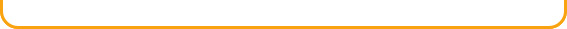Math HomepageMathLanguage ArtsReadingScienceSocial StudiesWorld AtlasExtra ToolsHomeJoinLoginBlogContact UsYou will need to receive a quiz score of at least 80% to complete each section. However, to get the most out of MyStudyBuddy.com, we recommended that you take quizzes as many times as necessary to achieve a quiz score of 100%.I'm Ed. I'll be your Study Buddy for math.  Choose a
topic from the list below.  Once you have found the topic
you would like to study, click on that link.Subtraction

Multiplication
Division
Word Problem
Solving
Roman Numerals

Exponents
Square Roots

Measurement

Scientific Notation
D​ecimals and
Operations
Introduction to
Fractions
Operations with
Fractions
Percents, Fractions,
and Decimals

Number Relationships
Operations with
Signed Numbers

Ratio & Rate

Application of
Proportion
Interest Problems
Probability
Graphs

One-Step Equations
Combining Like
Terms

Multi-Step Equations

One-Step
Inequalities

Polynomials
Ordered Pairs

Linear Equations
Equations

Distance Between
Two Points

Intercepts
Slope of a Line
Slope-Intercept
Form
Factoring by
Grouping

Introduction to
Geometry
Perimeter

Area

Circumference
V​olume
Angles
Triangles
Pythagorean
Theorem

Trigonometric
Ratios